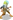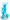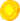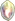## FANDOM

156 Pages

This page is a collection of formulas used for calculating various in-game numbers.

## Formulas

### Hero Cost Formula (cost of upgrading from "Level")

The cost to level upCid, the Helpful Adventurer from level 1 to 15 is:
floor((5 + Level) × 1.07Level - 1)
The cost to level upCid, the Helpful Adventurer from level 16 is:
floor(20 × 1.07Level - 1)
The cost to level up other hero by one is:
floor(BaseCost × 1.07Level - 1)

### Hero DPS

DPS of each hero, the number displayed on the right side of the hero's levelling buttons, is a product of

• Hero Base Damage
• Hero Level
• ×4 Multiplier for each 25 Hero Levels, starting from level 200; at Hero Level 1000, 2000, ..., 8000 the multiplier is ×10. Heroes afterFrostleaf have a ×5 multiplier instead of ×4 for each 25 Hero levels from 525 to 725;
• Personal DPS Upgrade bonuses
• ×2 Damage Bonus, which costs 50 rubies
• Dark Ritual Stacks (×1.05 with each normal DR, ×1.1 with each Energized DR)
• Global DPS Upgrades:
• ×1.25 fromThe Wandering Fisherman;
• ×2.0736 fromBetty Clicker;
• ×1.25 fromLeon;
• ×1.25 fromBroyle Lindeoven, Fire Mage;
• ×1.44 fromAmenhotep;
• ×1.1 fromBeastlord;
• ×1.1 fromShinatobe, Wind Deity;
• ×1.5625 fromGrant, The General;
• ×1.25 fromFrostleaf;
• ×1.5 fromGog;
• ×1.5 fromMoeru;
• ×2.34375 fromZilar;
• ×1.11 fromYachiyl
• Bonus from unusedHero Souls andMorgulis, which equals to (1 +Hero Souls × 0.1 + Level ofMorgulis × 0.11)
• Gild bonus, which is (1 + (0.5 + Level ofArgaiv × 0.02) × NumberOfGilds)
•Phandoryss bonus (+100% DPS per level)

Total DPS, the number displayed on the upper left side of Hero tab, is a product of:

• Sum of all Heroes DPS
• Bonus fromSiyalatas andNogardnit (idle mode)
• Bonus fromJuggernaut Combo (active mode)
• ×2 if Powersurge is activated (×3 with Energized Powersurge)

### Hero DPS perGold

All character level costs use the same multiplier (Base×1.07Level). They also have the same scaling WRT DPS / level. The only difference is their base cost to base DPS ratio, and personal modifiers to damage. For example,Treebeast cost 50 gold for level 1, but gives you 5 base DPS (10:1 ratio), whileFrostleaf costs 2.1e27 and gives 7.5e22 base DPS (28,113:1 ratio).

Below level 200, the scaling is:

DPS = BaseDPS × StaticModifier × Level

Cost = BaseCost × 1.07Level


StaticModifier includes the global adjustment, personal modifiers and gilding, but does not change based on the level of the character.

DPS/Cost = (BaseDPS*StaticModifier/BaseCost) × (Level/1.07Level) = Static × Level × 1.07-Level


(At level 199, this is Static × 199×(1.07-199) = Static × 2.828e-4, or 0.03% the DPS/gold you got at level 1).

After level 200, the 4Level term starts to dominate, so we can ignore the other terms. You get

DPS = Base×Static×Level×4Level/25~Constant×4Level/25=Constant × eln(4)/(25×L)=C×e^(0.0555 L)

DPS/Cost = Static×e^(0.0555 L)/(1.07^L) = Static×e^(0.0555 L)×e-0.0677L=Static×e-0.01222L=Static×0.988L=Static(1-0.012)L


So each level is 1.2% less efficient of a DPS increase vs the cost to do the increase.

Each Hero is a little less efficient than the previous one.Ivan, the Drunken Brawler being 14% less base efficient thanTreebeast, down to a low ofBroyle Lindeoven, Fire Mage being 189% less base efficient thanBobby, Bounty Hunter. AfterBroyle Lindeoven, Fire Mage, each one, up toFrostleaf, is a static 36% less efficient than the previous one. At a 25 level upgrade, this is 26.3% less efficient. Which is fairly close to the average difference between heroes. So ignoring personal modifiers, it is close to optimally efficient to level each hero 25 levels higher than the next hero.

###Hero Souls awarded for killing Primal BossesHero Souls awarded by defeating Primal Bosses:
floor(((Level - 80) / 25)1.3 × (1 + (Bonus from Ponyboy)))
Transcendent PowerHero Souls awarded by defeating Primal Bosses (only for transcendent player):
20 × (1 + (Bonus from Ponyboy)) × (1 + TP%)(Level / 5) - 20

Note: Omeet (Centurion Boss at Level 100) always gives 1Hero Souls and 0 Transcendent PowerHero Souls, regardless of the formulas above.

### Monster HP for levels

From Level 1 to Level 140:
$\left \lceil 10\times(Level-1+1.55^{Level-1})\times [isBoss\times 10] \right \rceil$
From Level 141 to Level 500:
$\left \lceil 10\times(139+1.55^{139}\times 1.145^{Level-140})\times [isBoss\times 10] \right \rceil$
From Level 501 to Level 200000:
$\left \lceil 10\times(139+1.55^{139}\times 1.145^{360}\times \prod_{i=501}^{Level}(1.145+0.001\times \left \lfloor \frac{i-1}{500} \right \rfloor))\times [isBoss\times 10] \right \rceil$
From Level 200001 onwards:
$\left \lceil (1.545^{Level-200001}\times 1.240\times 10^{25409}+((Level-1)\times 10)\right \rceil$

$\prod$ is the product symbol,

$\left \lceil \right \rceil$ are ceiling brackets and

$\left \lfloor \right \rfloor$ are flooring brackets.

### MonsterGold Drop

This is how muchGold you will get (on average) from killing a monster.Gold dropped is the product of:

• Monster gold worth (see below),
• 1 + 9 × (Fortuna Chance),
• Bonus fromLibertas (idle mode).

### Monster Gold Worth

This is how much killing a monster is "worth" - it is used in determiningGold dropped (see above) and for the Golden Clicks skill. Monster worth is the ceiling (round up) of the product of:

• Monster's HP / 15,
• Min(3, 1.025level - 75) if level is greater than 75,
•Gold Found Upgrades:
• × 2.9296875 fromKing Midas,
• × 1.5 fromBomber Max,
• × 3 fromTsuchi,
• Bonus fromMammon,
• × 10 × (Bonus fromMimzee), if it is aTreasure Chest,
• × 2 if Metal Detector is activated (×3 with Energized Metal Detector).

## Formula for sum of n1.5

The approximation below can be used to estimate the total cost of raising an Ancient with upgrade cost at level n equals to n1.5, from level 1 to level n:

$C(n)\approx\frac{2}{5}n^{\frac{5}{2}}+\frac{1}{2}n^{\frac{3}{2}}+\frac{1}{8}n^{\frac{1}{2}}+\frac{1}{1920}n^{-\frac{3}{2}}+O(n^{-\frac{5}{2}})+R$

where R≈-0.0254852. This result can be obtained with the Euler-Maclaurin formula. In practice, the terms after n1/2 can be ignored, leaving the following:

$C(n)\approx\frac{2}{5}n^{\frac{5}{2}}+\frac{1}{2}n^{\frac{3}{2}}+\frac{1}{8}n^{\frac{1}{2}}$ The cost to level such Ancient, from level x to level y, can be estimated using

$C(y)-C(x)$ .